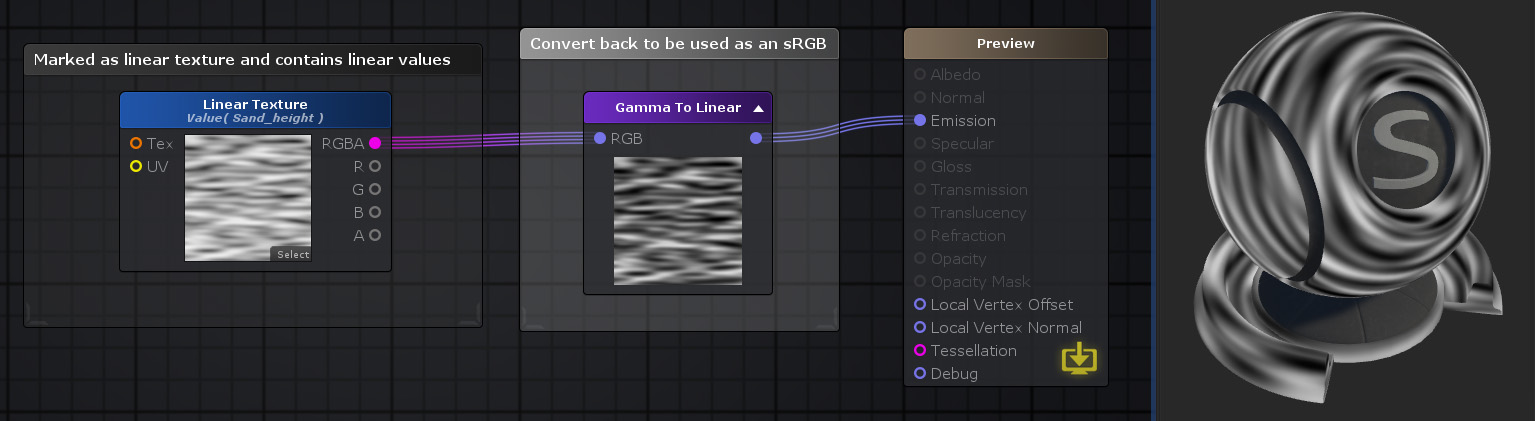# Unity Products:Amplify Shader Editor/Gamma To Linear

## Gamma To Linear Node

The Gamma To Linear node converts a color value in sRGB(Gamma) space to Linear space.
Through toggling On/Off the Exact Conversion property, its outputted result can be calculated by either using an exact conversion method or using a faster but less accurate approximation.Nodes used: Texture Sample, Gamma To Linear

Node Parameter Description Default Value
RGB Color value to be converted from sRGB(Gamma)to Linear space. Only visible if the respective input port is not connected. 0,0,0
Mode Switch between accurate color conversion method or faster but less accurate color conversion method.
• Fast sRGB to Linear: Fast approximation from sRGB to Linear
• Exact sRGB to Linear: A more expensive but exact calculation from sRGB to Linear
• Gamma 2.0 to Linear: Crude approximation from Gamma to Linear using a power of 2.0 gamma value
• Gamma 2.2 to Linear: An approximation from Gamma to Linear using a power of 2.2 gamma value
Fast sRGB to Linear

Input Port Description Type
RGB Color value to be converted from sRGB(Gamma) to Linear space. Float3